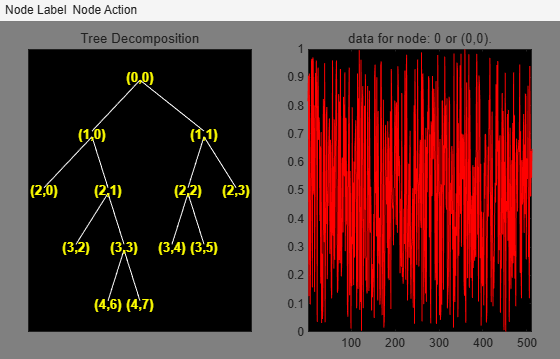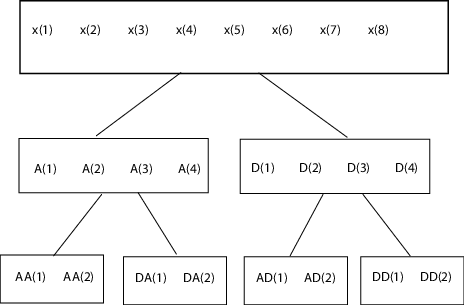Main Content

# otnodes

Order terminal nodes of binary wavelet packet tree

## Syntax

```[Tn_Pal,Tn_Seq] = otnodes(WPT) [Tn_Pal,Tn_Seq,I,J] = otnodes(WPT) [DP_Pal,DP_Seq] = otnodes(WPT,'dp') ```

## Description

```[Tn_Pal,Tn_Seq] = otnodes(WPT)``` returns the terminal nodes of the binary wavelet packet tree, `WPT`, in Paley (natural) ordering, `Tn_Pal`, and sequency (frequency) ordering, `Tn_Seq`. `Tn_Pal` and `Tn_Seq` are N-by-1 column vectors where N is the number of terminal nodes.

```[Tn_Pal,Tn_Seq,I,J] = otnodes(WPT)``` returns the permutations of the terminal node indices such that `Tn_Seq = Tn_Pal(I)` and ```Tn_Pal = Tn_Seq(J)```.

```[DP_Pal,DP_Seq] = otnodes(WPT,'dp')``` returns the Paley and frequency-ordered terminal nodes in node depth-position format. `DP_Pal` and `DP_Seq` are N-by-2 matrices. The first column contains the depth index, and the second column contains the position index.

## Input Arguments

 `WPT` Binary wavelet packet tree. You can use `treeord` to determine the order of your wavelet packet tree. `dp` Character vector indicating that the Paley-ordered or sequency-ordered nodes are returned in depth-position format.

## Output Arguments

 `Tn_Pal` Terminal nodes in Paley (natural) ordering `Tn_Seq` Terminal nodes in sequency ordering `DP_Pal` Paley-ordered terminal nodes in depth-position format. This output argument only applies when you use the `'dp'` input argument. `DP_Seq` Sequency-ordered terminal nodes in depth-position format. This output argument only applies when you use the `'dp'` input argument.

## Examples

collapse all

Order terminal nodes with Paley and frequency ordering.

```x = randn(8,1); wpt = wpdec(x,2,'haar'); [Tn_Pal,Tn_Seq] = otnodes(wpt)```
```Tn_Pal = 4×1 3 4 5 6 ```
```Tn_Seq = 4×1 3 4 6 5 ```

Return permutations for Paley and frequency ordering.

```load noisdopp; wpt = wpdec(noisdopp,6,'sym4'); [Tn_Pal,Tn_Seq,I,J] = otnodes(wpt); isequal(Tn_Seq(J),Tn_Pal)```
```ans = logical 1 ```
`isequal(Tn_Seq,Tn_Pal(I))`
```ans = logical 1 ```

Order terminal nodes by depth and position.

```x = randn(8,1); wpt = wpdec(x,2,'haar'); [DP_Pal,DP_Seq] = otnodes(wpt,'dp')```
```DP_Pal = 4×2 2 0 2 1 2 2 2 3 ```
```DP_Seq = 4×2 2 0 2 1 2 3 2 2 ```

Order terminal nodes from a modified wavelet packet tree.

```t = wptree(2,2,rand(1,512),'haar'); t = wpsplt(t,4); t = wpsplt(t,5); t = wpsplt(t,10); plot(t);```` [tn_Pal,tn_Seq,I,J] = otnodes(t)`
```tn_Pal = 7×1 3 9 21 22 11 12 6 ```
```tn_Seq = 7×1 3 21 22 9 6 12 11 ```
```I = 7×1 1 3 4 2 7 6 5 ```
```J = 7×1 1 4 2 3 7 6 5 ```

## More About

collapse all

### Paley (Natural) and Sequency (Frequency) Ordering

The discrete wavelet packet transform iterates on both approximation and detail coefficients at each level. In this transform, A denotes the lowpass (approximation) filter followed by downsampling. D denotes the highpass (detail) filter followed by downsampling. The following figure represents a wavelet packet transform in Paley ordering acting on a time series of length 8. The transform has a depth of two.Because of aliasing introduced by downsampling, the frequency content extracted by the operator AD is higher than the frequency content extracted by the DD operator. Therefore, the terminal nodes in frequency (sequency) order are: AA,DA,DD,AD. The terminal nodes in Paley order have the following indices: 3,4,5,6. The frequency order has the indices: 3,4,6,5.

## References

Wickerhauser, M.V. Lectures on Wavelet Packet Algorithms, Technical Report, Washington University, Department of Mathematics, 1992.

## See Also

Introduced in R2010b

## SupportGet trial now